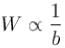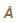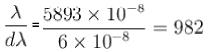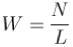Courses

# Diffraction MCQ Level - 1

## 10 Questions MCQ Test Topic wise Tests for IIT JAM Physics | Diffraction MCQ Level - 1

Description
This mock test of Diffraction MCQ Level - 1 for IIT JAM helps you for every IIT JAM entrance exam. This contains 10 Multiple Choice Questions for IIT JAM Diffraction MCQ Level - 1 (mcq) to study with solutions a complete question bank. The solved questions answers in this Diffraction MCQ Level - 1 quiz give you a good mix of easy questions and tough questions. IIT JAM students definitely take this Diffraction MCQ Level - 1 exercise for a better result in the exam. You can find other Diffraction MCQ Level - 1 extra questions, long questions & short questions for IIT JAM on EduRev as well by searching above.
QUESTION: 1

### What changes are observed in a diffraction pattern if the whole apparatus is immersed in water?

Solution:

Explanation: As the whole apparatus is now immersed in water, the wavelength of the light will change. λ’= λ / μ
Therefore, as the refractive index of water is greater than the air, the wavelength of light will decrease. Width of central maxima = 2λ / a
Therefore, as the wavelength decreases, the width of the central maxima decreases.

QUESTION: 2

### How does the width W of the central maximum formed from diffraction through a circular aperture (pupil) change with aperture size (b) for a fixed distance away from the aperture?

Solution:

Width of central maxima is inversely proportional to slit width or aperture size .The correct answer is: W decreases as b increases

QUESTION: 3

### A grating is able to resolve two very close spectral lines of wavelength 5890and 5896in its first order diffraction. The resolving power of the grating is :

Solution:

The two lines will be seen distinctly if they are resolved by grating.
Resolving power∴ The correct option is close to 1000.
The correct answer is: close to 1000

QUESTION: 4

The diffraction pattern due to circular aperture consists of :

Solution:

The correct answer is: a bright central disc surrounded by alternate dark and bright concentric rings

QUESTION: 5

The distance between the maxima of two wavelengths seen on a screen, after passing through a diffraction grating :

Solution:

dsin(theta) = m (lambda)

If distance between the maximas increases it means sin(theta) will increase so to normalize that d should decrease. It means that ‘d’ should decrease which in turn means that the number of slits per cm will increase.

QUESTION: 6

According to Rayleigh’s criterion of resolution, the two spectral lines of equal intensity are just resolved when the central maximum of the diffraction pattern due to one falls :

Solution:

The correct answer is: on the first minimum of the diffraction pattern of other

QUESTION: 7

Dispersive power of a grating can be defined as :

Solution:

The correct answer is: Increase of angle of diffraction w.r.t. change in wavelength

QUESTION: 8

The resolving power of a grating :

Solution:

The correct answer is: increases as the total number of lines on the grating increases

QUESTION: 9

Which one of the following plane transmission grating of width W, and number of lines per cm, L, will have the maximum resolving power in the first order?

Solution:

Resolving power = nN, where n is order of diffraction and N is the total number of ruling on grating.
N = WL
∴ R.P  = nWLwhere n = 1
R.P= 1 x 5000 = 5000
R.P= 1.5 x 4000 = 6000
R.P= 2 x 2400 = 4800
R.P= 3 x 1500 = 4500
The correct answer is: W = 1.5 cm, L = 4000

QUESTION: 10

If N is the total number of rulings on the grating, m is the order of spectrum and A is the wavelength of light used, then resolving power of grating is given by :

Solution: